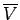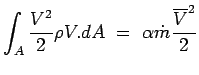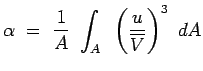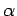## Kinetic Energy Correction Factor

We have assumed in the derivation of Bernoulli equation that the velocity at the end sections (1) and (2) is uniform. But in a practical situation this may not be the case and the velocity can very across the cross section. A remedy is to use a correction factor for the kinetic energy term in the equation. Ifis the average velocity at an end section then we can write for energy,(3.75)

After simplification we find that(3.76)

Consequently, Eqn.3.70 (Low Speed Application) is written as(3.77)

whereis the Kinetic Energy Factor. Its value for a fully developed laminar pipe flow is around 2, whereas for a turbulent pipe flow it is between 1.04 to 1.11. It is usual to take it is 1 for a turbulent flow. It should not be neglected for a laminar flow.

(c) Aerospace, Mechanical & Mechatronic Engg. 2005
University of Sydney